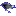# functions

This category contains 18 nodes.

##### GaussianProcesses (3.6)

Implements Gaussian Processes for regression without hyperparameter-tuning. For more information see David J.C. Mackay (1998). Introduction to Gaussian […]

##### IsotonicRegression (3.6)

Learns an isotonic regression model. Picks the attribute that results in the lowest squared error. Missing values are not allowed. Can only deal with […]

##### LeastMedSq (3.6)

Implements a least median sqaured linear regression utilising the existing weka LinearRegression class to form predictions. Least squared regression […]

##### LibLINEAR (3.6)

A wrapper class for the liblinear tools (the liblinear classes, typically the jar file, need to be in the classpath to use this classifier). Rong-En Fan, […]

##### LibSVM (3.6)

A wrapper class for the libsvm tools (the libsvm classes, typically the jar file, need to be in the classpath to use this classifier). LibSVM runs faster […]

##### LinearRegression (3.6)

Class for using linear regression for prediction. Uses the Akaike criterion for model selection, and is able to deal with weighted instances.

##### Logistic (3.6)

Class for building and using a multinomial logistic regression model with a ridge estimator. There are some modifications, however, compared to the paper […]

##### MultilayerPerceptron (3.6)

A Classifier that uses backpropagation to classify instances. This network can be built by hand, created by an algorithm or both. The network can also be […]

##### PaceRegression (3.6)

Class for building pace regression linear models and using them for prediction. Under regularity conditions, pace regression is provably optimal when the […]

##### PLSClassifier (3.6)

A wrapper classifier for the PLSFilter, utilizing the PLSFilter's ability to perform predictions.